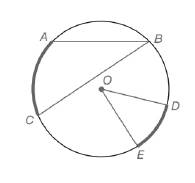Chapter 6.1, Problem 1E### Elementary Geometry for College St...

6th Edition
Daniel C. Alexander + 1 other
ISBN: 9781285195698

#### Solutions

Chapter
Section### Elementary Geometry for College St...

6th Edition
Daniel C. Alexander + 1 other
ISBN: 9781285195698
Textbook Problem
67 views

# For Exercises 1 to 8, use the figure provided.Exercises 1-8If m A C ⌢ = 58 ∘ ,  find m ∠ B .

To determine

To calculate:

The mB, by using the provided figure.

Explanation

Given:

The provided figure is given below:

Theorem used:

The measure of an inscribed angle of a circle is one-half the measure of its intercepted arc.

Calculation:

Given, mAC=58o.

Since, the measure of an inscribed angle of a circle is one-half the measure of its intercepted arc.

mB=12mAC

### Still sussing out bartleby?

Check out a sample textbook solution.

See a sample solution

#### The Solution to Your Study Problems

Bartleby provides explanations to thousands of textbook problems written by our experts, many with advanced degrees!

Get Started

#### Explain what happens during each of the two stages of the two-factor ANOVA.

Essentials of Statistics for The Behavioral Sciences (MindTap Course List)

#### True or False: These lines are skew:

Study Guide for Stewart's Multivariable Calculus, 8th

#### For what values of p does the series converge?

Study Guide for Stewart's Single Variable Calculus: Early Transcendentals, 8th

#### Define the validity of measurement and explain why and how it is measured.

Research Methods for the Behavioral Sciences (MindTap Course List)

#### Distinguish between a hypothesis and a prediction.

Research Methods for the Behavioral Sciences (MindTap Course List)